# Suslin hypothesis

(diff) ← Older revision | Latest revision (diff) | Newer revision → (diff)

A hypothesis that states that every linearly ordered set without first and last elements that is moreover complete, dense and satisfies the Suslin condition, is isomorphic to the real line. Here, completeness signifies the existence of a least upper bound for every non-empty bounded subset, density denotes the non-emptiness of any interval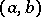,, and the Suslin condition requires that every family of pairwise-disjoint intervals is countable. The real line possesses all the properties that figure in the formulation of the Suslin hypothesis. The Suslin hypothesis thus states that the above properties of an ordered set define it completely. This hypothesis was formulated in 1920 by M.Ya. Suslin .

Within the framework of the system ZFC (the system ZF with the axiom of choice) it is impossible to prove or disprove the Suslin hypothesis, assuming that ZF is consistent. It follows from Gödel's axiom of constructibility (see Gödel constructive set) that the negation of the Suslin hypothesis holds. The consistency of the Suslin hypothesis with the axioms of ZFC is proved by the construction of a corresponding model, using a variant of the forcing method (iterated forcing). The addition of the continuum hypothesis to ZFC does not give either a positive or negative solution of the Suslin hypothesis.

The Suslin hypothesis and its generalizations have had a great influence on the development of axiomatic set theory. Many ideas and methods have been worked out in conjunction with it. These include Jensen's combinatorial principles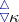and(see ), the theory of the fine structure of the constructible hierarchy (see ), Martin's axiom , and the iterated forcing method .

Jensen's principle: A subset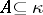of a cardinal number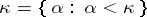is said to be closed unbounded if it contains all its limit points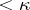and if, for any, there is a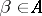such that. A setis said to be stationary if its intersection with every closed unbounded subset of a cardinal numberis non-empty.

Jensen's principle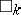: There exists a sequence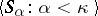,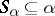, such that for every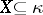the set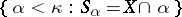is stationary. For every regular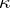, the principlefollows from the axiom of constructibility, while the negation of the Suslin hypothesis follows from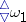. Jensen's combinatorial principles, as well as Martin's axiom (see below), have been successfully used in topology (see , , ).

Letbe a partially ordered set. A set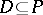is called dense if for everythere is asuch that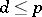. A set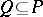is said to be compatible if for any finite subset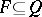there is a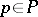such that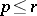for every. Two elementsand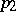fromare said to be incompatible if the set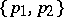is not compatible. It is said that a partially ordered setsatisfies the countable anti-chain condition if every set that consists of pairwise-incompatible elements is countable. Martin's axiom (MA) states the following: If a partially ordered setsatisfies the countable anti-chain condition and if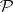is a family of dense subsets of cardinality less than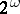, then there is a compatible setsuch that for everythe intersectionis non-empty.

In the presence of the continuum hypothesis (CH), Martin's axiom can be proved. The most interesting results are obtained by a combination of Martin's axiom (MA) and the negation of the continuum hypothesis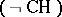. The principle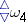contradicts the combination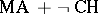, sinceimplies CH. It often turns out that a result that can be inferred fromwill be disproved under the assumption. This is, e.g., the case with the Suslin hypothesis. Indeed,implies the Suslin hypothesis, whileimplies the negation of the Suslin hypothesis.

The combinationis compatible with ZFC if ZF is consistent.

How to Cite This Entry:
Suslin hypothesis. Encyclopedia of Mathematics. URL: http://encyclopediaofmath.org/index.php?title=Suslin_hypothesis&oldid=18257
This article was adapted from an original article by V.N. Grishin (originator), which appeared in Encyclopedia of Mathematics - ISBN 1402006098. See original article